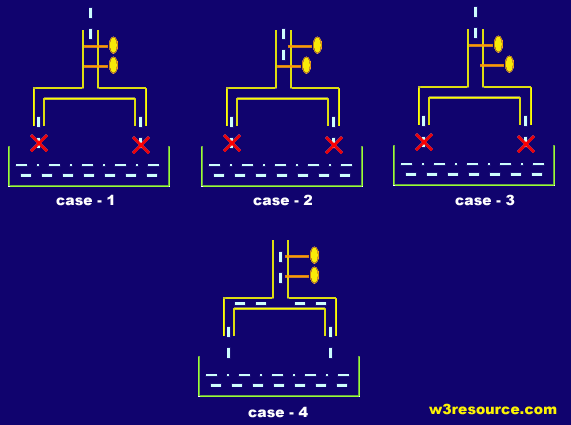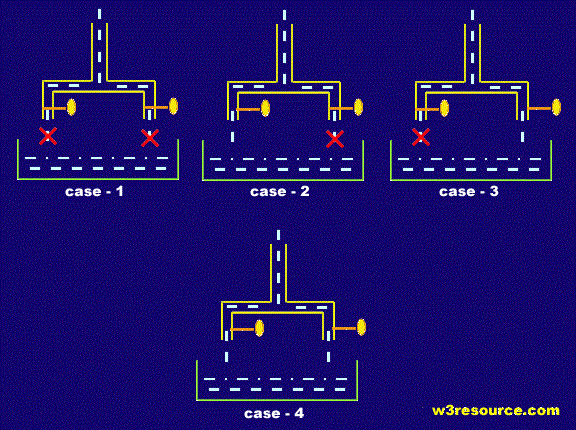# Ruby Logical Operators

## Logical Operators

The standard logical operators and, or and not are supported by Ruby. Logical operators first convert their operands to boolean values and then perform the respective comparison.

Logical and :

The binary "and" operator returns the logical conjunction of its two operands. The condition becomes true if both the operands are true. It is the same as "&&" but with a lower precedence. See the following presentation :This above pictorial helps you to understand the concept of LOGICAL AND operation with an analogy of taps and water.

In case-1 of the picture, both of the taps are closed, so the water is not flowing down. Which explains that if both of conditions are FALSE or 0, the return is FALSE or 0.

In case-2 of the picture, one of the taps are closed, even then, the water is not flowing down. Which explains that even if any of conditions are FALSE or 0, the return is FALSE or 0.

case-3 of the picture resembles CASE -2.

In case-4 of the picture, both of the taps are open, so the water is flowing down. Which explains that if both of conditions are TRUE or 1, the return is TRUE or 1.

So we can conclude that if and only if, both of the conditions are TRUE or 1, LOGICAL AND operations returns TRUE or 1.

Logical or :

The binary "or" operator returns the logical disjunction of its two operands. The condition becomes true if both the operands are true. It is the same as "||" but with a lower precedence. See the following presentation:The above pictorial helps you to understand the concept of LOGICAL OR operation with an analogy of taps and water.

In case-1 of the picture, both of the taps are closed, so the water is not flowing down. Which explains that if both of conditions are FALSE or 0, the return is FALSE or 0.

In case-2 of the picture, one of the taps are closed, and we can see that the water is flowing down. Which explains that if any of conditions are TRUE or 1, the return is TRUE or 1.

case-3 of the picture, resembles CASE -2.

In case-4 of the picture, both of the taps are open, so the water is flowing down. Which explains that if both of conditions are TRUE or 1, the return is TRUE or 1.

So we can conclude that in LOGICAL OR operation, if any of the conditions are true, the output is TRUE or 1.

Logical not:

The logical not or ! operator is used to reverse the logical state of its operand. If a condition is false, the logical not operator makes it true.

Example: Ruby logical operator

`````` puts ("logical operators in Ruby")
ruby = "x"
programming = "y"
if ruby == "foo" && programming == "bar"
puts "&&"
end

if ruby == "foo" and programming == "bar"
puts "&& and"
end

p, q, r, s = 1, 2 ,3 , 4
if p == 1 && q == 2 && r == 3 && s == 4
puts sum = p + q + r + s
end

programming = "ruby"

if ruby == "foo" || programming == "bar"
puts "||"
end

if ruby == "foo" or programming == "bar"
puts "|| or"
end

ruby = "awesome"
if ruby == "foo" or programming == "bar"
puts "|| or"
else
puts "sorry!"
end

if not (ruby == "foo" || programming == "bar")
puts "nothing!"
end

if !(ruby == "foo" or programming == "bar")
puts "nope!"
end
``````

Output:

```logical operators in Ruby
10
sorry!
nothing!
nope!
```

Example-1: Ruby operators (&&, and) precedence

``````irb(main):016:0> foo = :foo
=> :foo
irb(main):017:0> bar = nil
=> nil
irb(main):018:0> x = foo and bar
=> nil
irb(main):019:0> x
=> :foo
irb(main):020:0> x = foo && bar
=> nil
irb(main):021:0> x
=> nil
irb(main):022:0> x = (foo and bar)
=> nil
irb(main):023:0> x
=> nil
irb(main):024:0> (x = foo) && bar
=> nil
irb(main):025:0> x
=> :foo
``````

Example-1: Ruby operators (||, or) precedence

``````irb(main):001:0> foo = :foo
=> :foo
irb(main):002:0> bar = nil
=> nil
irb(main):003:0> x = foo or bar
=> :foo
irb(main):004:0> x
=> :foo
irb(main):005:0> x = foo || bar
=> :foo
irb(main):006:0> x
=> :foo
irb(main):007:0> x = (foo or bar)
=> :foo
irb(main):008:0> x
=> :foo
irb(main):009:0> (x = foo) || bar
=> :foo
irb(main):010:0> x
=> :foo
``````

Previous: Ruby Bitwise Operatorsent
Next: Ruby Ternary operator

﻿